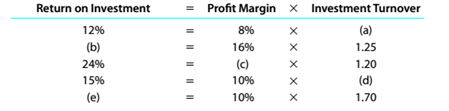Chapter 14, Problem 14.12E

Chapter
Section
Textbook Problem

Determining missing items in return on investment computationsOne item is omitted from each of the following computations of the return on investmentDetermine the missing items, identifying each by the appropriate letter.

To determine

Concept Introduction:

Profit Margins: It is a measure which is generally used by the organization to find out the best department in the organization in terms of profit. To calculate the profit margins divide the profits with sales.

Return on investment: In this we divide the total return or profit with the investment into the assets of organization. It is the best way of calculating the % of return in the business.

To Calculate:

Find out the missing figure?

Explanation

a. = ROI/ Profit margin

= 12%/8%

= 1.5

b. = Profit margin* Investment Turnover

= 16%* 1.25

= 20%

c. = ROI/ Investment Turnover

= 24%/ 1...

Still sussing out bartleby?

Check out a sample textbook solution.

See a sample solution

The Solution to Your Study Problems

Bartleby provides explanations to thousands of textbook problems written by our experts, many with advanced degrees!

Get Started

AFTER-TAX COST OF DEBT The Heuser Companys currently outstanding bonds have a 10% coupon and a 12% yield to mat...

Fundamentals of Financial Management, Concise Edition (with Thomson ONE - Business School Edition, 1 term (6 months) Printed Access Card) (MindTap Course List)

Should an economic model describe reality exactly?

Essentials of Economics (MindTap Course List)

What is a composite primary key?

Pkg Acc Infor Systems MS VISIO CD